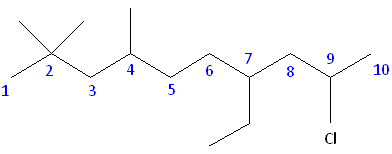# 4.1: How Alkyl Substituents Are Named

The names of all alkanes end with -ane. Whether or not the carbons are linked together end-to-end in a ring (called cyclic alkanes or cycloalkanes) or whether they contain side chains and branches, the name of every carbon-hydrogen chain that lacks any double bonds or functional groups will end with the suffix -ane.

Alkanes with unbranched carbon chains are simply named by the number of carbons in the chain. The first four members of the series (in terms of number of carbon atoms) are named as follows:

1. CH4 = methane = one hydrogen-saturated carbon
2. C2H6 = ethane = two hydrogen-saturated carbons
3. C3H8 = propane = three hydrogen-saturated carbons
4. C4H10 = butane = four hydrogen-saturated carbons

Alkanes with five or more carbon atoms are named by adding the suffix -ane to the appropriate numerical multiplier, except the terminal -a is removed from the basic numerical term. Hence, C5H12 is called pentane, C6H14 is called hexane, C7H16 is called heptane and so forth.

Straight-chain alkanes are sometimes indicated by the prefix n- (for normal) to distinguish them from branched-chain alkanes having the same number of carbon atoms. Although this is not strictly necessary, the usage is still common in cases where there is an important difference in properties between the straight-chain and branched-chain isomers: e.g. n-hexane is a neurotoxin while its branched-chain isomers are not.

IUPAC nomenclature

The IUPAC nomenclature is a system on which most organic chemists have agreed to provide guidelines to allow them to learn from each others' works. Nomenclature, in other words, provides a foundation of language for organic chemistry.

## Number of Hydrogen to Carbons

This equation describes the relationship between the number of hydrogen and carbon atoms in alkanes:

H = 2C + 2

where "C" and "H" are used to represent the number of carbon and hydrogen atoms present in one molecule. If C = 2, then H = 6.

Many textbooks put this in the following format:

CnH2n+2

where "Cn" and "H2n+2" represent the number of carbon and hydrogen atoms present in one molecule. If Cn = 3, then H2n+2 = 2(3) + 2 = 8. (For this formula look to the "n" for the number, the "C" and the "H" letters themselves do not change.)

Progressively longer hydrocarbon chains can be made and are named systematically, depending on the number of carbons in the longest chain.

The following table contains the systematic names for the first twenty straight chain alkanes. It will be important to familiarize yourself with these names because they will be the basis for naming many other organic molecules throughout your course of study.

### Drawing Hydrocarbons

Recall that when carbon makes four bonds, it adopts the tetrahedral geometry. In the tetrahedral geometry, only two bonds can occupy a plane simultaneously. The other two bonds point in back or in front of this plane. In order to represent the tetrahedral geometry in two dimensions, solid wedges are used to represent bonds pointing out of the plane of the drawing toward the viewer, and dashed wedges are used to represent bonds pointing out of the plane of the drawing away from the viewer. Consider the following representation of the molecule methane:Figure 1: Two dimensional representation of methane

In the above drawing, the two hydrogens connected by solid lines, as well as the carbon in the center of the molecule, exist in a plane (specifically, the plane of the computer monitor / piece of paper, etc.). The hydrogen connected by a solid wedge points out of this plane toward the viewer, and the hydrogen connected by the dashed wedge points behind this plane and away from the viewer.

In drawing hydrocarbons, it can be time-consuming to write out each atom and bond individually. In organic chemistry, hydrocarbons can be represented in a shorthand notation called a skeletal structure. In a skeletal structure, only the bonds between carbon atoms are represented. Individual carbon and hydrogen atoms are not drawn, and bonds to hydrogen are not drawn. In the case that the molecule contains just single bonds (sp3 bonds), these bonds are drawn in a "zig-zag" fashion. This is because in the tetrahedral geometry all bonds point as far away from each other as possible, and the structure is not linear. Consider the following representations of the molecule propane: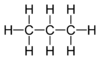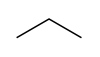Figure 2: Full structure of propane and skeletal structure of propane

Only the bonds between carbons have been drawn, and these have been drawn in a "zig-zag" manner. Note that there is no representation of hydrogens in a skeletal structure. Since, in the absence of double or triple bonds, carbon makes four bonds total, the presence of hydrogens is implicit. Whenever an insufficient number of bonds to a carbon atom are specified in the structure, it is assumed that the rest of the bonds are made to hydrogens. For example, if the carbon atom makes only one explicit bond, there are three hydrogens implicitly attached to it. If it makes two explicit bonds, there are two hydrogens implicitly attached, etc. Note also that two lines are sufficient to represent three carbon atoms. It is the bonds only that are being drawn out, and it is understood that there are carbon atoms (with three hydrogens attached!) at the terminal ends of the structure.

### Alkyl Groups

Alkanes can be described by the general formula CnH2n+2. An alkyl group is formed by removing one hydrogen from the alkane chain and is described by the formula CnH2n+1. The removal of this hydrogen results in a stem change from -ane to -yl. Take a look at the following examples.The same concept can be applied to any of the straight chain alkane names provided in the table above.

Name Molecular Formula Condensed Structural Formula
Methane CH4 CH4
Ethane C2H6 CH3CH3
Propane C3H8 CH3CH2CH3
Butane C4H10 CH3(CH2)2CH3
Pentane C5H12 CH3(CH2)3CH3
Hexane C6H14 CH3(CH2)4CH3
Heptane C7H16 CH3(CH2)5CH3
Octane C8H18 CH3(CH2)6CH3
Nonane C9H20 CH3(CH2)7CH3
Decane C10H22 CH3(CH2)8CH3
Undecane C11H24 CH3(CH2)9CH3
Dodecane C12H26 CH3(CH2)10CH3
Tridecane C13H28 CH3(CH2)11CH3
Eicosane C20H42 CH3(CH2)18CH3

### Using Common Names with Branched Alkanes

Certain branched alkanes have common names that are still widely used today. These common names make use of prefixes, such as iso-, sec-, tert-, and neo-. The prefix iso-, which stands for isomer, is commonly given to 2-methyl alkanes. In other words, if there is methyl group located on the second carbon of a carbon chain, we can use the prefix iso-. The prefix will be placed in front of the alkane name that indicates the total number of carbons. Examples:

• isopentane which is the same as 2-methylbutane
• isobutane which is the same as 2-methylpropane

To assign the prefixes sec-, which stands for secondary, and tert-, for tertiary, it is important that we first learn how to classify carbon molecules. If a carbon is attached to only one other carbon, it is called a primary carbon. If a carbon is attached to two other carbons, it is called a seconday carbon. A tertiary carbon is attached to three other carbons and last, a quaternary carbon is attached to four other carbons. Examples:

• 4-sec-butylheptane (30g)
• 4-tert-butyl-5-isopropylhexane (30d); if using this example, may want to move sec/tert after iso disc

The prefix neo- refers to a substituent whose second-to-last carbon of the chain is trisubstituted (has three methyl groups attached to it). A neo-pentyl has five carbons total. Examples:

• neopentane
• neoheptane

### Alkoxy Groups

Alkoxides consist of an organic group bonded to a negatively charged oxygen atom. In the general form, alkoxides are written as RO-, where R represents the organic substituent. Similar to the alkyl groups above, the concept of naming alkoxides can be applied to any of the straight chain alkanes provided in the table above.### Three Principles of Naming

1. Choose the longest, most substituted carbon chain containing a functional group.
2. A carbon bonded to a functional group must have the lowest possible carbon number. If there are no functional groups, then any substitute present must have the lowest possible number.
3. Take the alphabetical order into consideration; that is, after applying the first two rules given above, make sure that your substitutes and/or functional groups are written in alphabetical order.

Example 1

What is the name of the following molecule?SOLUTION

Rule #1: Choose the longest, most substituted carbon chain containing a functional group. This example does not contain any functional groups, so we only need to be concerned with choosing the longest, most substituted carbon chain. The longest carbon chain has been highlighted in red and consists of eight carbons.Rule #2: Carbons bonded to a functional group must have the lowest possible carbon number. If there are no functional groups, then any substitute present must have the lowest possible number. Because this example does not contain any functional groups, we only need to be concerned with the two substitutes present, that is, the two methyl groups. If we begin numbering the chain from the left, the methyls would be assigned the numbers 4 and 7, respectively. If we begin numbering the chain from the right, the methyls would be assigned the numbers 2 and 5. Therefore, to satisfy the second rule, numbering begins on the right side of the carbon chain as shown below. This gives the methyl groups the lowest possible numbering.Rule 3: In this example, there is no need to utilize the third rule. Because the two substitutes are identical, neither takes alphabetical precedence with respect to numbering the carbons. This concept will become clearer in the following examples.

Example 2

What is the name of the following molecule?SOLUTION

Rule #1: Choose the longest, most substituted carbon chain containing a functional group. This example contains two functional groups, bromine and chlorine. The longest carbon chain has been highlighted in red and consists of seven carbons.Rule #2: Carbons bonded to a functional group must have the lowest possible carbon number. If there are no functional groups, then any substitute present must have the lowest possible number. In this example, numbering the chain from the left or the right would satisfy this rule. If we number the chain from the left, bromine and chlorine would be assigned the second and sixth carbon positions, respectively. If we number the chain from the right, chlorine would be assigned the second position and bromine would be assigned the sixth position. In other words, whether we choose to number from the left or right, the functional groups occupy the second and sixth positions in the chain. To select the correct numbering scheme, we need to utilize the third rule.Rule #3: After applying the first two rules, take the alphabetical order into consideration. Alphabetically, bromine comes before chlorine. Therefore, bromine is assigned the second carbon position, and chlorine is assigned the sixth carbon position.Example 3

What is the name of the follow molecule?SOLUTION

Rule #1: Choose the longest, most substituted carbon chain containing a functional group. This example contains two functional groups, bromine and chlorine, and one substitute, the methyl group. The longest carbon chain has been highlighted in red and consists of seven carbons.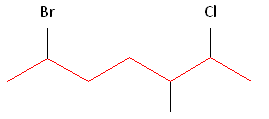Rule #2: Carbons bonded to a functional group must have the lowest possible carbon number. After taking functional groups into consideration, any substitutes present must have the lowest possible carbon number. This particular example illustrates the point of difference principle. If we number the chain from the left, bromine, the methyl group and chlorine would occupy the second, fifth and sixth positions, respectively. This concept is illustrated in the second drawing below. If we number the chain from the right, chlorine, the methyl group and bromine would occupy the second, third and sixth positions, respectively, which is illustrated in the first drawing below. The position of the methyl, therefore, becomes a point of difference. In the first drawing, the methyl occupies the third position. In the second drawing, the methyl occupies the fifth position. To satisfy the second rule, we want to choose the numbering scheme that provides the lowest possible numbering of this substitute. Therefore, the first of the two carbon chains shown below is correct.Therefore, the first numbering scheme is the appropriate one to use.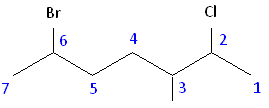Once you have determined the correct numbering of the carbons, it is often useful to make a list, including the functional groups, substitutes, and the name of the parent chain.

Rule #3: After applying the first two rules, take the alphabetical order into consideration. Alphabetically, bromine comes before chlorine. Therefore, bromine is assigned the second carbon position, and chlorine is assigned the sixth carbon position.

Parent chain: heptane 2-Chloro 3-Methyl 6-Bromo

6-bromo-2-chloro-3-methylheptane

### Problems

What is the name of the follow molecules?